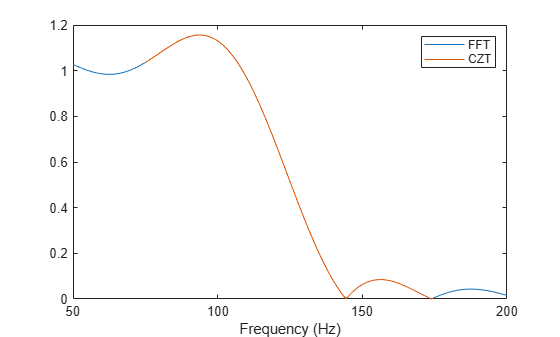Main Content

# czt

Chirp Z-transform

## Syntax

``y = czt(x,m,w,a)``

## Description

example

````y = czt(x,m,w,a)` returns the length-`m` chirp Z-transform (CZT) of `x` along the spiral contour on the z-plane defined by `w` and `a` through ```z = a*w.^-(0:m-1)```.With the default values of `m`, `w`, and `a`, `czt` returns the Z-transform of `x` at `m` equally spaced points around the unit circle, a result equivalent to the discrete Fourier transform (DFT) of `x` as given by `fft(x)`.```

## Examples

collapse all

Create a random vector, `x`, of length 1013. Compute its DFT using `czt`.

```rng default x = randn(1013,1); y = czt(x);```

Use `czt` to zoom in on a narrow-band section of a filter's frequency response.

Design a 30th-order lowpass FIR filter using the window method. Specify a sample rate of 1 kHz and a cutoff frequency of 125 Hz. Use a rectangular window. Find the transfer function of the filter.

```fs = 1000; d = designfilt('lowpassfir','FilterOrder',30,'CutoffFrequency',125, ... 'DesignMethod','window','Window',@rectwin,'SampleRate',fs); h = tf(d);```

Compute the DFT and the CZT of the filter. Restrict the frequency range of the CZT to the band between 75 and 175 Hz. Generate 1024 samples in each case.

```m = 1024; y = fft(h,m); f1 = 75; f2 = 175; w = exp(-j*2*pi*(f2-f1)/(m*fs)); a = exp(j*2*pi*f1/fs); z = czt(h,m,w,a);```

Plot the transforms. Zoom in on the area of interest.

```fn = (0:m-1)'/m; fy = fs*fn; fz = (f2-f1)*fn + f1; plot(fy,abs(y),fz,abs(z)) xlim([50 200]) legend('FFT','CZT') xlabel('Frequency (Hz)')```## Input Arguments

collapse all

Input signal, specified as a vector, a matrix, or a 3-D array. If `x` is a matrix, the function transforms the columns of `x`. If `x` is a 3-D array, the function operates along the first array dimension with size greater than 1.

Example: `sin(pi./[4;2]*(0:159))'` specifies a two-channel sinusoid.

Data Types: `single` | `double`
Complex Number Support: Yes

Transform length, specified as a positive integer scalar.

Data Types: `single` | `double`

Ratio between spiral contour points, specified as a complex scalar.

Data Types: `single` | `double`
Complex Number Support: Yes

Spiral contour initial point, specified as a complex scalar.

Example: `exp(1j*pi/4)` lies along the unit circle on the z-plane and makes an angle of 45 degrees with the real axis.

Data Types: `single` | `double`
Complex Number Support: Yes

## Output Arguments

collapse all

Chirp Z-transform, returned as a vector or matrix.

## Algorithms

`czt` uses the next power-of-2 length FFT to perform a fast convolution when computing the Z-transform on a specified chirp contour .

 Rabiner, Lawrence R., and Bernard Gold. Theory and Application of Digital Signal Processing. Englewood Cliffs, NJ: Prentice-Hall, 1975.

## Support

#### Deep Learning for Signal Processing with MATLAB

Download white paper